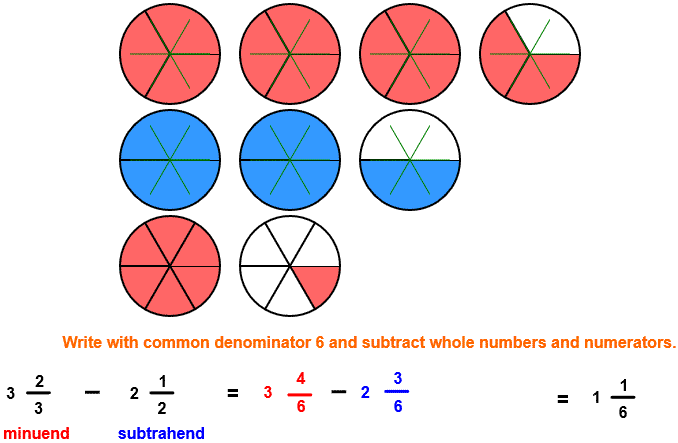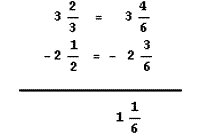# Subtract Fractions

## Subtract and Simplify Fractions with Circle Models

SUBTRACT UNLIKE FRACTIONS
CORRECT:
ATTEMPTS:
SCORE:
PERCENT
WHOLE
NUMERATOR
DENOMINATOR

# SUBTRACT FRACTIONS INSTRUCTIONS

Follow the directions in the dialog box after pressing the <START> button. The <EXPLAIN> button may be pressed after you enter the subtrahend to see how to do the example.

The following image was made by Subtract Fractions with Circles Designer:The picture shows that the minuend is 3 23 units and the subtrahend is 2 12 units. The difference is what is left after removing the blue circles in the second row from the red circles in the first row. In many examples, you can arrive at the difference by looking at the picture. For example, after removing the two whole blue circles and the 12 circle in the minuend from the subtrahend , you are left with 76 or 1 16 circles.

When the program starts, you will be asked to identify the minuend in the first row and then the subtrahend in the second row. The program will continue when the minuend and subtrahend are correctly identified. You will then be asked to find the difference. The difference must be entered as a whole number or as a mixed number in lowest terms.

You may prefer to work vertically:For more instruction on identifying mixed fractions go to How To Rename From Fraction Form to Mixed Form.

For more instruction on renaming to lower terms go to How To Rename to Lower Terms.

For more instruction on subtracting fractions go to How To Subtract Fractions.

After you enter the difference you may press the <REPORT> button. The report will ask for your name but you may submit a code for your name. This report will give the same results as on the dialog box. The report may be printed or e-mailed.